# Section 6.3. Stream Ciphers and RC4

[Page 189 (continued)]

### 6.3. Stream Ciphers and RC4

In this section we look at perhaps the most popular symmetric stream cipher, RC4. We begin with an overview of stream cipher structure, and then examine RC4.

#### Stream Cipher Structure

A typical stream cipher encrypts plaintext one byte at a time, although a stream cipher may be designed to operate on one bit at a time or on units larger than a byte at a time. Figure 6.8 is a representative diagram of stream cipher structure. In this structure a key is input to a pseudorandom bit generator that produces a stream of 8-bit numbers that are apparently random. We discuss pseudorandom number generators in Chapter 7. For now, we simply say that a pseudorandom stream is one that is unpredictable without knowledge of the input key. The output of the generator, called a keystream, is combined one byte at a time with the plaintext stream using the bitwise exclusive-OR (XOR) operation. For example, if the next byte generated by the generator is 01101100 and the next plaintext byte is 11001100, then the resulting ciphertext byte is

[Page 190]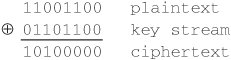Decryption requires the use of the same pseudorandom sequence: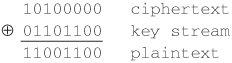##### Figure 6.8. Stream Cipher Diagram (This item is displayed on page 189 in the print version)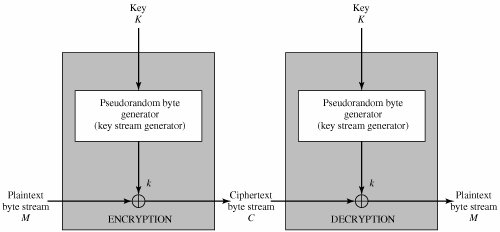The stream cipher is similar to the one-time pad discussed in Chapter 2. The difference is that a one-time pad uses a genuine random number stream, whereas a stream cipher uses a pseudorandom number stream.

[KUMA97] lists the following important design considerations for a stream cipher:

1. The encryption sequence should have a large period. A pseudorandom number generator uses a function that produces a deterministic stream of bits that eventually repeats. The longer the period of repeat the more difficult it will be to do cryptanalysis. This is essentially the same consideration that was discussed with reference to the Vigenère cipher, namely that the longer the keyword the more difficult the cryptanalysis.

2. The keystream should approximate the properties of a true random number stream as close as possible. For example, there should be an approximately equal number of 1s and 0s. If the keystream is treated as a stream of bytes, then all of the 256 possible byte values should appear approximately equally often. The more random-appearing the keystream is, the more randomized the ciphertext is, making cryptanalysis more difficult.

3. Note from Figure 6.8 that the output of the pseudorandom number generator is conditioned on the value of the input key. To guard against brute-force attacks, the key needs to be sufficiently long. The same considerations as apply for block ciphers are valid here. Thus, with current technology, a key length of at least 128 bits is desirable.

With a properly designed pseudorandom number generator, a stream cipher can be as secure as block cipher of comparable key length. The primary advantage of a stream cipher is that stream ciphers are almost always faster and use far less code than do block ciphers. The example in this section, RC4, can be implemented in just a few lines of code. Table 6.2, using data from [RESC01], compares execution times of RC4 with three well-known symmetric block ciphers. The advantage of a block cipher is that you can reuse keys. However, if two plaintexts are encrypted with the same key using a stream cipher, then cryptanalysis is often quite simple [DAWS96]. If the two ciphertext streams are XORed together, the result is the XOR of the original plaintexts. If the plaintexts are text strings, credit card numbers, or other byte streams with known properties, then cryptanalysis may be successful.

[Page 191]

##### Table 6.2. Speed Comparisons of Symmetric Ciphers on a Pentium II

Cipher

Key Length

Speed (Mbps)

DES

56

9

3DES

168

3

RC2

variable

0.9

RC4

variable

45

For applications that require encryption/decryption of a stream of data, such as over a data communications channel or a browser/Web link, a stream cipher might be the better alternative. For applications that deal with blocks of data, such as file transfer, e-mail, and database, block ciphers may be more appropriate. However, either type of cipher can be used in virtually any application.

#### The RC4 Algorithm

RC4 is a stream cipher designed in 1987 by Ron Rivest for RSA Security. It is a variable key-size stream cipher with byte-oriented operations. The algorithm is based on the use of a random permutation. Analysis shows that the period of the cipher is overwhelmingly likely to be greater than 10100 [ROBS95a]. Eight to sixteen machine operations are required per output byte, and the cipher can be expected to run very quickly in software. RC4 is used in the SSL/TLS (Secure Sockets Layer/Transport Layer Security) standards that have been defined for communication between Web browsers and servers. It is also used in the WEP (Wired Equivalent Privacy) protocol and the newer WiFi Protected Access (WPA) protocol that are part of the IEEE 802.11 wireless LAN standard. RC4 was kept as a trade secret by RSA Security. In September 1994, the RC4 algorithm was anonymously posted on the Internet on the Cypherpunks anonymous remailers list.

The RC4 algorithm is remarkably simply and quite easy to explain. A variable-length key of from 1 to 256 bytes (8 to 2048 bits) is used to initialize a 256-byte state vector S, with elements S, S,..., S. At all times, S contains a permutation of all 8-bit numbers from 0 through 255. For encryption and decryption, a byte k (see Figure 6.8) is generated from S by selecting one of the 255 entries in a systematic fashion. As each value of k is generated, the entries in S are once again permuted.

##### Initialization of S

To begin, the entries of S are set equal to the values from 0 through 255 in ascending order; that is; S = 0, S = 1,..., S = 255. A temporary vector, T, is also created. If the length of the key K is 256 bytes, then K is transferred to T. Otherwise, for a key of length keylen bytes, the first keylen elements of T are copied from K and then K is repeated as many times as necessary to fill out T. These preliminary operations can be summarized as follows:

[Page 192]

`/* Initialization */ for  i = 0 to 255 do S[i] = i; T[i] = K[i mod keylen]; `

Next we use T to produce the initial permutation of S. This involves starting with S and going through to S, and, for each S[i], swapping S[i] with another byte in S according to a scheme dictated by T[i]:

`/* Initial Permutation of S */ j = 0; for  i = 0 to 255 do   j = (j + S[i] + T[i]) mod 256;   Swap (S[i], S[j]); `

Because the only operation on S is a swap, the only effect is a permutation. S still contains all the numbers from 0 through 255.

##### Stream Generation

Once the S vector is initialized, the input key is no longer used. Stream generation involves cycling through all the elements of S[i], and, for each S[i], swapping S[i] with another byte in S according to a scheme dictated by the current configuration of S. After S is reached, the process continues, starting over again at S:

`/* Stream Generation */ i, j = 0; while (true)   i = (i + 1) mod 256;   j = (j + S[i]) mod 256;   Swap (S[i], S[j]);   t = (S[i] + S[j]) mod 256;   k = S[t]; `

To encrypt, XOR the value k with the next byte of plaintext. To decrypt, XOR the value k with the next byte of ciphertext.

Figure 6.9 illustrates the RC4 logic.

##### Figure 6.9. RC4 (This item is displayed on page 193 in the print version)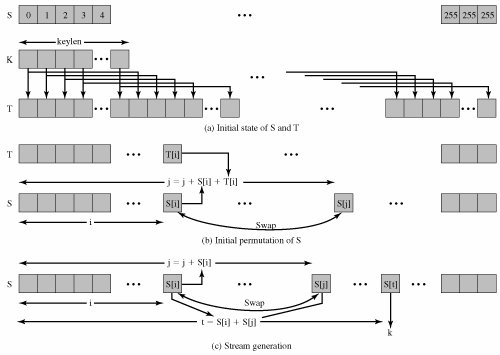##### Strength of RC4

A number of papers have been published analyzing methods of attacking RC4 [e.g., [KNUD98], [MIST98], [FLUH00], [MANT01]). None of these approaches is practical against RC4 with a reasonable key length, such as 128 bits. A more serious problem is reported in [FLUH01]. The authors demonstrate that the WEP protocol, intended to provide confidentiality on 802.11 wireless LAN networks, is vulnerable to a particular attack approach. In essence, the problem is not with RC4 itself but the way in which keys are generated for use as input to RC4. This particular problem does not appear to be relevant to other applications using RC4 and can be remedied in WEP by changing the way in which keys are generated. This problem points out the difficulty in designing a secure system that involves both cryptographic functions and protocols that make use of them.

[Page 194]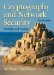Cryptography and Network Security (4th Edition)
ISBN: 0131873164
EAN: 2147483647
Year: 2005
Pages: 209